## A single tiny logic gate

The first case we’ll look at is a Fairchild NC7SZ04 ultra high speed inverter gate.  This logic gate is a single inverter gate in a SOT-23 package and is part of a Picotest demonstration board designed to illustrate a PDN issue in a very simple low power circuit.  The inverter gate is used as a buffer between a 10MHz clock and a 50 Ohm port.  The output impedance of the logic gate is approximately 20Ω and R20 adds an additional 30Ω Ohms to total 50Ω in order to match the coaxial cable and input terminator on the oscilloscope.  Resistor R18 is a 0805 package film resistor, which was increased from 0.2 Ω (as shown in Figure 2) to 1Ω in the tests here to make the device current signal easier to see and so the scaling is 1V/A.  This resistor does contribute to the issue, but it is not the dominant term.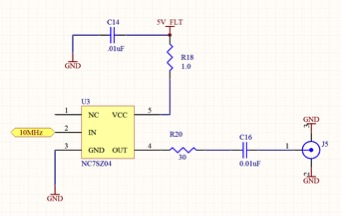Figure 1 Schematic of the logic gate buffer circuit, which converts the high output impedance, 10MHz clock to a 50Ω output.

The very small section of the demonstration PCB that includes this logic gate is shown in Figure 2.  The section shown is approximately 1.2” wide top provide some sense of scale.  The decoupling capacitor for the logic gate, C14 is connected to the groundplane on the top side and three approximately 1/8” traces connect the capacitor to the 1 Ω resistor and to the Vcc pin of the logic gate.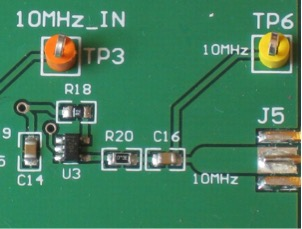Figure 2 This small section of the board shows the logic gate, U3 along with the decoupling cap, C14 the current sense resistor, R18 the output coupling resistor, R20 and the AC coupling capacitor, C16.

The first thing that many will find surprising is that the edge speed of the logic gate is approximately 400ps as shown in Figure 3. This picture also shows the AC voltage across the decoupling capacitor using a 4GHz oscilloscope and a 4GHz active probe.  The probe and scope combination have a rise time of approximately 125ps and so this measurement is not limited by the measurement bandwidth.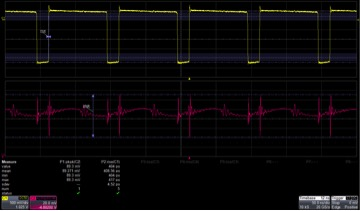Figure 3 The output of the logic gate (top trace) and the AC voltage across the decoupling capacitor C14 (bottom trace)

The peak to peak voltage at the decoupling capacitor is 89mV, which for a 5V part is not unreasonable.  The voltage at the logic gate supply voltage pins is shown in Figure 4.Figure 4 The AC coupled voltage at the pins of the logic gate using the same oscilloscope and probe pairing.

The peak to peak voltage at the logic gate has increased to more than 1V, which is far beyond the maximum recommended range for most 5V devices.  This particular part has a very wide operating range of 1.65V-5.5V.  With a nominal 5V input this voltage still comes very close to the maximum operating voltage.

A 1.5GHz differential voltage was used to measure the voltage across the 1Ω resistor and the frequency spectrum of the voltage.  The measurement is shown in Figure 5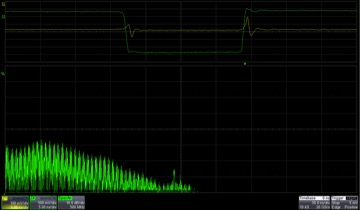Figure 5 Voltage across the 1Ω resistor (yellow trace) and voltage spectrum (bottom green trace) using a 1.5GHz differential probe and a 4GHz oscilloscope

The very narrow current pulse and fast edge speed means that it is likely that the scope and probe combination are insufficient with the 1.5GHz differential probe.  The same measurement is performed with a 20GHz oscilloscope paired with a 13GHz differential probe and the result is shown in Figure 6.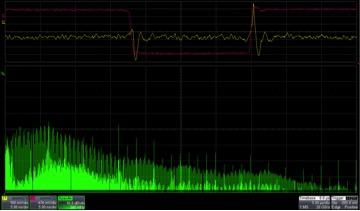Figure 6 Voltage across the 1Ω resistor (yellow trace) and voltage spectrum (bottom green trace) using a 13GHz differential probe and a 20GHz oscilloscope shows frequency content above 4GHz.

The peak to peak voltage is distributed between many elements, some resistive and some inductive.  Since there is no groundplane under these traces the expected inductance is estimated at 20nH/inch.

 Element Characteristic Inductive Resistive Estimated Inductance Decoupling cap Mostly inductive 89mVpp 600pH estimated Decoupling cap to 1Ω resistor Mostly inductive 569mVpp 5nH (0.25 in) 1Ω resistor Mostly resistive 700mV - 1Ω resistor to Vcc pin Mostly inductive 289mV 2.5nH (0.125”) Totals 947mV 700mV 8.5nH

The various signal levels are added using root sum squares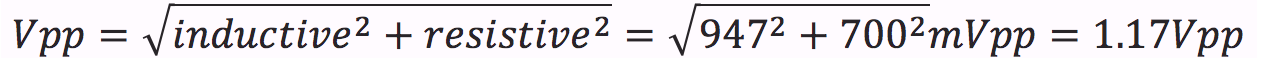(1)

And this simple calculation is in very good agreement with the measured result in Figure 3.

Another impact of the inductance is that these inductive traces radiate EMI.   Looking at the traces with a near field H probe close to the inductive traces as shown in Figure 7.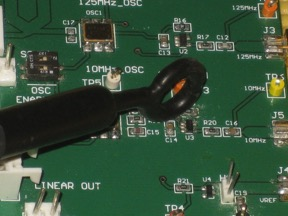Figure 7 Setup image showing small 1GHz near field prove at the trace connecting the decoupling capacitor to the 1Ω resistor.

And the frequency spectrum shows the rich radiated spectral content.  The near field probe starts to fall off at about 1GHz and so the harmonics likely extend out to about 5GHz as seen in the voltage across the 1Ω sense resistor.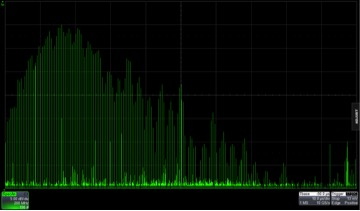Figure 8 The trace inductance between the decoupling capacitor and the 1Ω resistor has a rich EMI signature as seen in the near field probe.

We have seen that the edge speed of a very high speed CMOS logic gate can create harmonics to 5GHz or more.  The 1Ω sense resistor allows us to get an indication of the transition energy of the device, though it does contribute to the peak to peak voltage.  Eliminating the resistor would reduce the peak to peak voltage by approximately 220mV.  There are several ways to easily improve this issue.  Of course several can be selected as defined by the performance required.

1.     Of course the 1Ω sense resistor should be removed.  It was only added in order to show the device transition current.

2.     The decoupling capacitor should be moved to the right side of the chip very close to pin 7 (Vcc) of the logic gate

3.     The capacitor ground should be connected to a bottom side ground plane under the device.

4.     Use a low ESL ceramic decoupling capacitor and/or two parallel ceramic capacitors.

5.     Switch to a multilayer PCB to reduce the distance between the signal trace and the groundplane.